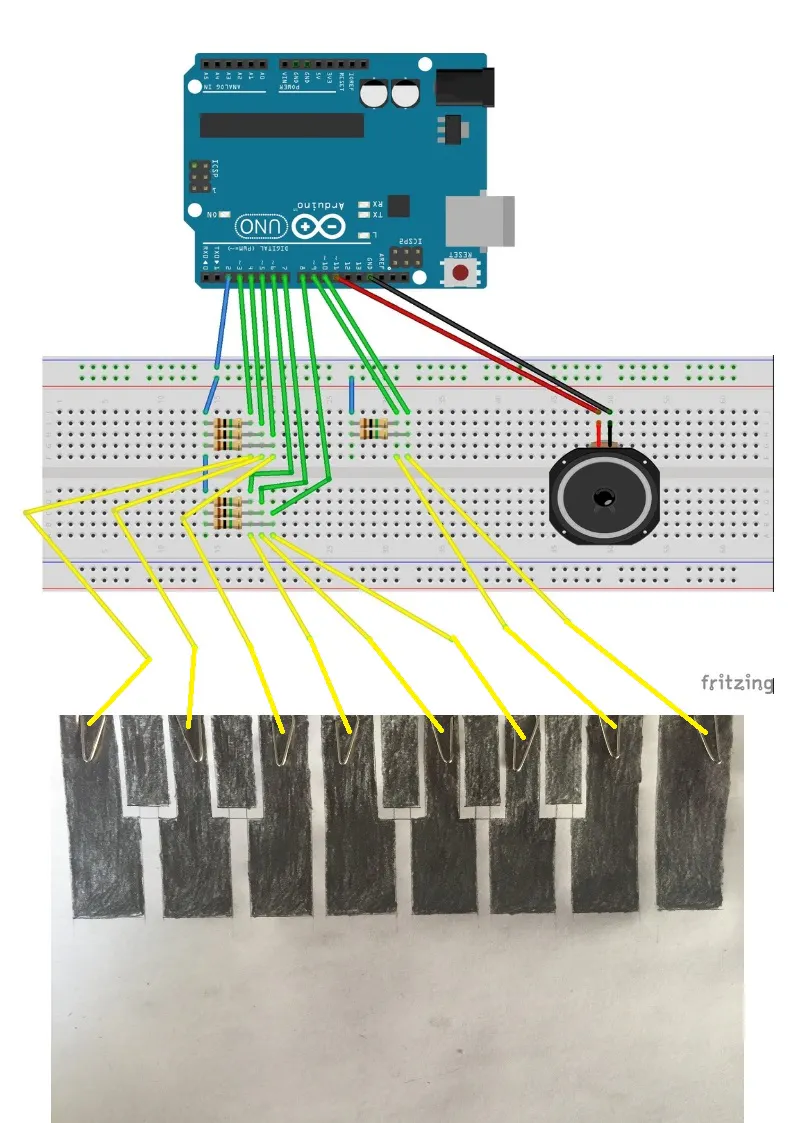# Paper Piano with Arduino

This project uses an Arduino, a drawn keyboard using lead pencil, a paper, and a speaker.

BeginnerShowcase (no instructions)6 hours83,665

## Things used in this project

### Hardware components

 Male-to-male jumper wires
×1
×1
 Arduino
×1
 Resistor 1M ohm
×1
 Speaker
×1

## Schematics

### Paper Piano## Code

### Paper_piano.ino

Arduino
```/* Original project by Oh Hai Seng 07 November 2013
To watch the Demo of his Project:

*/

// Import the CapacitiveSensor Library.
#include <CapacitiveSensor.h>

#define speaker 11

// Set the Send Pin & Receive Pin.
CapacitiveSensor   cs_2_3 = CapacitiveSensor(2,3);
CapacitiveSensor   cs_2_4 = CapacitiveSensor(2,4);
CapacitiveSensor   cs_2_5 = CapacitiveSensor(2,5);
CapacitiveSensor   cs_2_6 = CapacitiveSensor(2,6);
CapacitiveSensor   cs_2_7 = CapacitiveSensor(2,7);
CapacitiveSensor   cs_2_8 = CapacitiveSensor(2,8);
CapacitiveSensor   cs_2_9 = CapacitiveSensor(2,9);
CapacitiveSensor   cs_2_10 = CapacitiveSensor(2,10);

void setup()
{
cs_2_6.set_CS_AutocaL_Millis(0xFFFFFFFF);     // turn off autocalibrate on channel 1 - just as an example

// Arduino start communicate with computer.
Serial.begin(9600);
}

void loop()
{
// Set a timer.
long start = millis();

// Set the sensitivity of the sensors.
long total1 =  cs_2_3.capacitiveSensor(3000);
long total2 =  cs_2_4.capacitiveSensor(3000);
long total3 =  cs_2_5.capacitiveSensor(3000);
long total4 =  cs_2_6.capacitiveSensor(3000);
long total5 =  cs_2_7.capacitiveSensor(3000);
long total6 =  cs_2_8.capacitiveSensor(3000);
long total7 =  cs_2_9.capacitiveSensor(3000);
long total8 =  cs_2_10.capacitiveSensor(3000);

Serial.print(millis() - start);        // check on performance in milliseconds
Serial.print("\t");                    // tab character for debug windown spacing

Serial.print(total1);                  // print sensor output 1
Serial.print("\t");                    // Leave some space before print the next output
Serial.print(total2);                  // print sensor output 2
Serial.print("\t");                    // Leave some space before print the next output
Serial.print(total3);                  // print sensor output 3
Serial.print("\t");                    // Leave some space before print the next output
Serial.print(total4);                  // print sensor output 4
Serial.print("\t");                    // Leave some space before print the next output
Serial.print(total5);                  // print sensor output 5
Serial.print("\t");                    // Leave some space before print the next output
Serial.print(total6);                  // print sensor output 6
Serial.print("\t");                    // Leave some space before print the next output
Serial.print(total7);                   // print sensor output 7
// Leave some space before print the next output
Serial.print("\t");
Serial.println(total8);                 // print sensor output 8
// "println" - "ln" represent as "line", system will jump to next line after print the output.

// When hand is touched the sensor, the speaker will produce a tone.
// I set a threshold for it, so that the sensor won't be too sensitive.
if (total1 > 500) tone(speaker,131);   // frequency
if (total2 > 500) tone(speaker,147);   // you can see https://www.arduino.cc/en/Tutorial/toneMelody if you want to change frequency
if (total3 > 500) tone(speaker,165);
if (total4 > 500) tone(speaker,175);
if (total5 > 500) tone(speaker,196);
if (total6 > 500) tone(speaker,220);
if (total7 > 500) tone(speaker,247);
if (total8 > 500) tone(speaker,262);

// When hand didn't touch on it, no tone is produced.
if (total1<=500  &  total2<=500  &  total3<=500 & total4<=500  &  total5<=500  &  total6<=500 &  total7<=500 &  total8<=500)
noTone(speaker);

delay(10);                             // arbitrary delay to limit data to serial port
}
```

## Credits

### Barqunics

5 projects • 70 followers
Hello, My name is Ikhsan